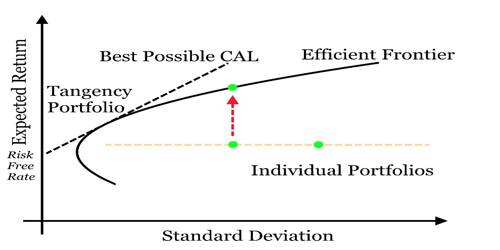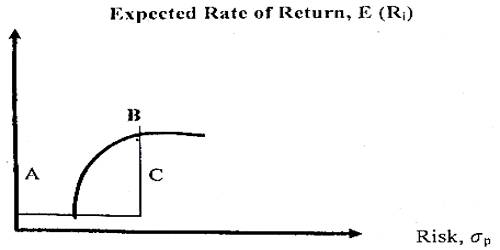# Efficient Frontier

Efficient Frontier

The efficient frontier represents that set of portfolios that have the maximum rate of return for every given level of risk, or the minimum risk or the minimum risk for every level of return. It is a method of analyzing a portfolio to discover the combination of assets that will produce the most return for risk. Every portfolio that lies on the efficient frontier has either a higher rate return for equal risk or lower risk for an equal rate of return than some portfolio beneath the frontier. It is the set of optimal portfolios that offers the highest expected return for a defined level of risk or the lowest risk for a given level of expected return. It is some referred to as the global mean-variance portfolio.Portfolios to the left of the efficient frontier are not possible because they lie outside the attainable set. Portfolios to the right of the efficient frontier are inefficient because some other portfolio would provide either a higher return with the same degree of risk or lower risk for the same rate of return. In the above figure portfolio ‘A’ dominates portfolio ‘C’ because it has an equal rate of return but substantially less risk. Similarly, portfolio ‘B’ dominates portfolio ‘C’ because it has an equal risk but a higher expected rate of return. Indeed portfolio C is dominated by all portfolios along curve AB because each one has the same or less risk while providing a greater or equal return. The efficient frontier is based on standard deviation, a measure of total risk.